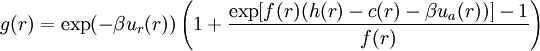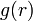# HMSA$g(r) = \exp(-\beta u_r(r)) \left(1+\frac{\exp[f(r)(h(r)-c(r)-\beta u_a(r))]-1}{f(r)}\right)$
where$g(r)$ is the radial distribution function.